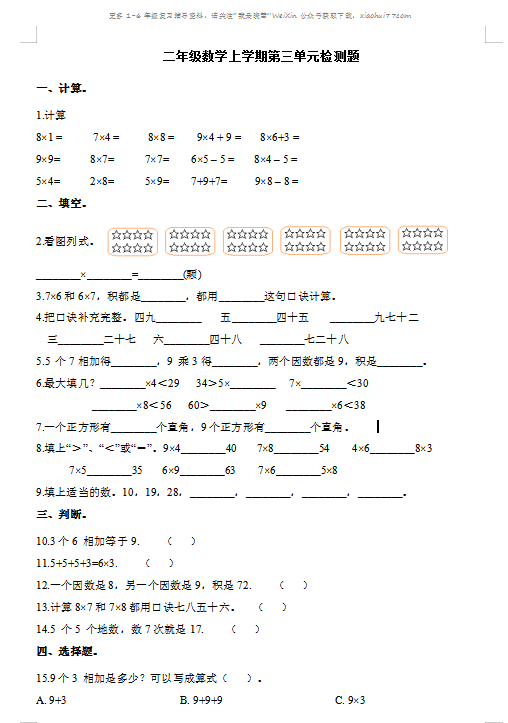1.计算

8×1 =          7×4 =         8×8 =       9×4 + 9 =      8×6+3 =
9×9=          8×7=          7×7=       6×5 – 5 =      8×4 – 5 =
5×4=          2×8=          5×9=       7+9+7=         9×8 – 8 =